Test: Logic Gates - 1

# Test: Logic Gates - 1

Test Description

## 10 Questions MCQ Test Digital Electronics | Test: Logic Gates - 1

Test: Logic Gates - 1 for Electrical Engineering (EE) 2023 is part of Digital Electronics preparation. The Test: Logic Gates - 1 questions and answers have been prepared according to the Electrical Engineering (EE) exam syllabus.The Test: Logic Gates - 1 MCQs are made for Electrical Engineering (EE) 2023 Exam. Find important definitions, questions, notes, meanings, examples, exercises, MCQs and online tests for Test: Logic Gates - 1 below.
Solutions of Test: Logic Gates - 1 questions in English are available as part of our Digital Electronics for Electrical Engineering (EE) & Test: Logic Gates - 1 solutions in Hindi for Digital Electronics course. Download more important topics, notes, lectures and mock test series for Electrical Engineering (EE) Exam by signing up for free. Attempt Test: Logic Gates - 1 | 10 questions in 30 minutes | Mock test for Electrical Engineering (EE) preparation | Free important questions MCQ to study Digital Electronics for Electrical Engineering (EE) Exam | Download free PDF with solutions
 1 Crore+ students have signed up on EduRev. Have you?
Test: Logic Gates - 1 - Question 1

### The output of the logic circuit shown in figure will be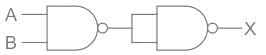Detailed Solution for Test: Logic Gates - 1 - Question 1

Concept:
Logic gate: The digital circuit that can be analyzed with the help of Boolean algebra is called a logic gate or logic circuit. A logic gate has two or more inputs but only one output.
The gate given below is NAND gate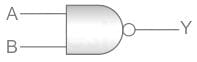NAND gate: It a combination of an AND and a NOT gate.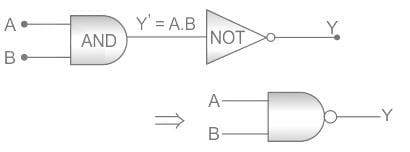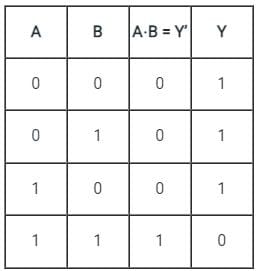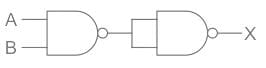From the first NAND Gate, the output will be (A⋅B)'
Now there will be 2 input of (A⋅B)' output will be [(A⋅B)']' which will give A⋅B as the correct answer.

Test: Logic Gates - 1 - Question 2

###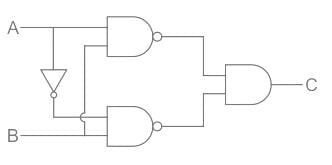The truth table for the given logic circuit is:

Detailed Solution for Test: Logic Gates - 1 - Question 2

Concept:
Here we have used concept of AND, and NAND gate concepts.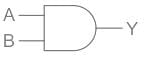If two inputs A, B are connected in And gate then the output can be written as: Y = A.B   ----- (1)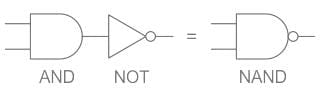In the case of the NAND gate when we connect two inputs A and b then output is: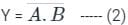Calculation:
Given: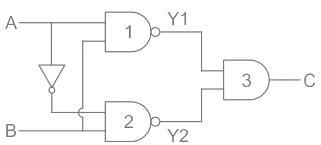Here in this circuit, we have labeled it as 1,2,3 part of circuit having outputs Y1, Y2, and C.
Y1 = solution of first two input NAND gate, Y2 = solution of second two input NAND gate
C = solution of third AND gate connecting both NAND gates as two inputs
From the circuit diagram, we can see that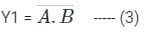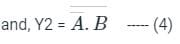Here output C = Y2.Y1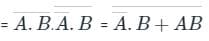(By De Morgan's theorem)
This can be written as: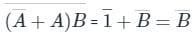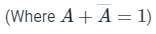So, we can write the truth table as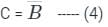when B = 0 then C = 1, B = 1 then C = 0 so that solution is completely independent on A.
So, the truth table is: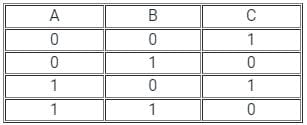Hence option 4) is correct.

Test: Logic Gates - 1 - Question 3

### For the logic circuit shown, the truth table is :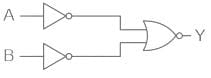Detailed Solution for Test: Logic Gates - 1 - Question 3

CONCEPT:
" NOT " gate - " NOT " gate is the logic gate in which input and output data are swapped. For example, if we are having input is 0 then its output is 1 and vice versa.
" NOR " gate - " NOR " gate is the type of logic gate and it is made up of the " OR " gate and the " NOT " gate.
CALCULATION: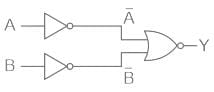In the above figure, we have two NOT gates after the input of A and B and after that, we have a NOR gate.
" NOT " gate is represented as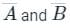and " NOR " gate of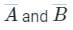is represented as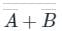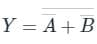Using the boolean algebra rule we have;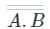⇒  A.B
⇒ AND Gate
Truth Table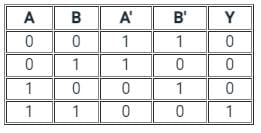Hence, Option 3) is the correct answer.

Test: Logic Gates - 1 - Question 4

The truth table for two input logic gate is as given below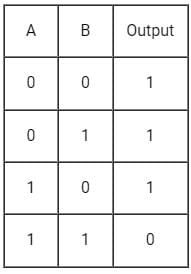Then the logic gate is

Detailed Solution for Test: Logic Gates - 1 - Question 4

CONCEPT:
Logic Gates:

• The logic gates are used in electronic circuits that give a certain output for a given set of Inputs.
• and which is based on a certain logic.
• Input and output are in binary form that is 1 and 0.
• It is based on certain Logic.
• Some basic logic gates are AND gate, OR gate, NOT gate.
• To build digital logic we develop a combination of these gates.

AND Gate:

• If both the inputs are high, it produces a high output.
• The Boolean algebra for AND gate is X = A. B
• It means that if any of the input of A and B is zero, the output will be zero.
• If both are one, the output will be one.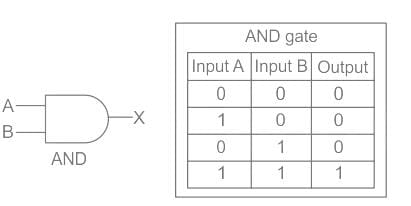NOT gate:

• It inverts the input. Whatever the input is given, it changes its value at the output.
• The Boolean algebra for NOT gate is X = X̅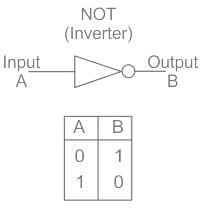NAND Gate:

• The NAND Gate is formed by the combination of AND gate and NOT gate.
• The output obtained from AND Gate is simply reversed.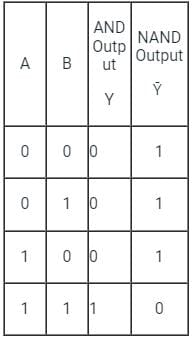• The output of the NAND Gate is similar to the output in the question.

So, the correct option is NAND Gate.

Test: Logic Gates - 1 - Question 5

Which logic gate will produce the following output?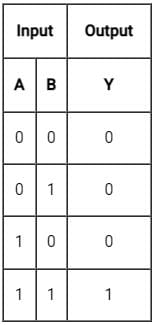Detailed Solution for Test: Logic Gates - 1 - Question 5

CONCEPT
Logic gates:

• It is an electric circuit, which works on simple Boolean algebra to perform a logical operation for one or more binary inputs that produce a single binary output.

Types of Logic gates:
AND Gate: If both the inputs are high, it produces a high output.

• The Boolean algebra for AND gate is X = A. B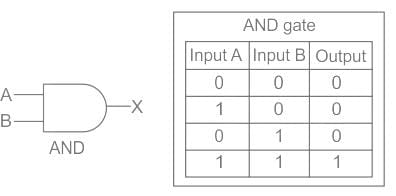And NAND gate is opposite of AND gate which means output is one when any of input is 1 whereas if both inputs is 1 output is 0
OR gate: If any of the input is high, it produces a high output.

• The Boolean algebra for OR gate is X = A + B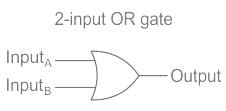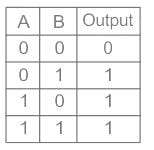NOT gate: It inverts the input. Whatever the input is given, it changes its value at the output.

• The Boolean algebra for NOT gate is X = X̅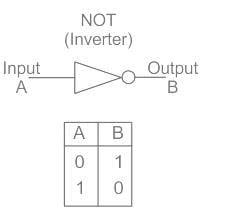From the above explanation, we can see that in our case the output for a given truth table is only possible for AND gate.

Test: Logic Gates - 1 - Question 6

The basic gates are:

Detailed Solution for Test: Logic Gates - 1 - Question 6
• AND, OR, NOT gates are the basic gates.
• The logic gates which are derived from the basic gates like AND, OR, NOT gates are known as derived gates. NAND, NOR, XOR, and XNOR are the derived gates.
• A universal gate is a gate which can implement any Boolean function without need to use any other gate type. The NAND and NOR gates are universal gates.
Test: Logic Gates - 1 - Question 7

The output of an OR gate is connected to both the inputs of a NAND gate. The combination will serve as

Detailed Solution for Test: Logic Gates - 1 - Question 7

CONCEPT:

• AND Gate: The Logic AND Gate is a digital logic circuit whose output is 1 (HIGH) only when all the inputs are 1 (HIGH)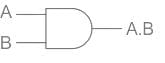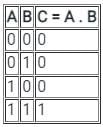• OR Gate: The Logic OR Gate is a digital logic circuit whose output goes HIGH to a logic level 1 only when one or more of its inputs are HIGH

​​​​​​​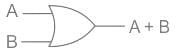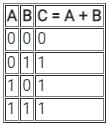• NOT Gate: The Logic NOT Gate is the most basic of all the logic gates and is often referred to as an Inverting Buffer or simply an Inverter.

​​​​​​​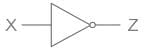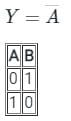• NAND Gate: The logic Gate is obtained after adding NOT Gate after AND Gate.

​​​​​​​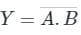• NOR Gate: The logic Gate is obtained after adding NOT Gate after OR Gate.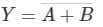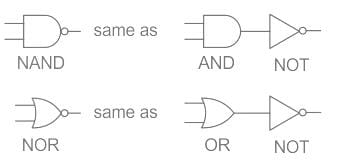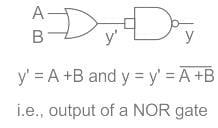• Since the output of OR Gate is input for both the inputs of NAND Gates, Both the inputs will be the same.
• When a NAND gate is connected to the same input it acts as a NOT Gate.
• The output of NAND Gate will be (same input)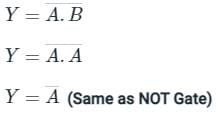• If NOT Gate is added after OR Gate, It will act as NOR Gate.
• So, The output of an OR gate is connected to both the inputs of a NAND gate. The combination will serve as NOR Gate. Hence the correct answer is option 4.
Test: Logic Gates - 1 - Question 8

Identify the logic gate?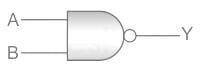Detailed Solution for Test: Logic Gates - 1 - Question 8

CONCEPT:

• Logic gate: The digital circuit that can be analyzed with the help of Boolean algebra is called a logic gate or logic circuit.
• A logic gate has two or more inputs but only one output.

NAND gate: It a combination of an AND and a NOT gate.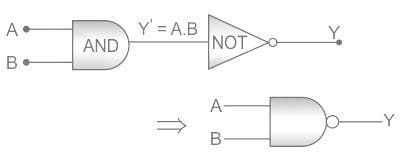It is obtained by connecting the output fo an AND gate to the input of a NOT gate.
It is described by the Boolean expression: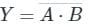The above logic gate is the NAND gate.
The truth table of NAND gate: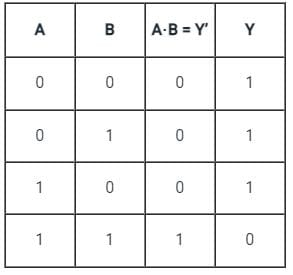Test: Logic Gates - 1 - Question 9

Which logic gate is equivalent to these combinations of logic gates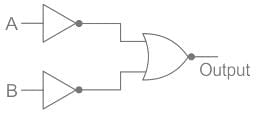Detailed Solution for Test: Logic Gates - 1 - Question 9

CONCEPT:
Logic gates are small electronic circuits that are used to control the output current according to our requirements.
These are the following symbols to represent logic gates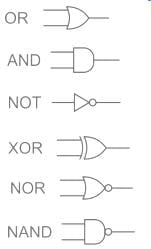These are the Properties of logic gates:
And gate(.) – It gives us an output one only when both the inputs as 1 otherwise 0
OR gate(+) – The OR gate gives an output of 1 if either of the two inputs is 1, it gives 0 otherwise.
NOT gate(‘) – The NOT gate gives an output of 1 input is 0 and vice-versa.
NOR gate – The combination of NOT and OR gates, so it will have its output reversed.
NAND gate – The combination of NOT and AND gates, so it will have its output reversed.
In our logic circuit, we used NOT and NOR in such a way that current from both A and B will pass through NOT gates and then send to the NOR gate hence the Truth table of the following circuit will be: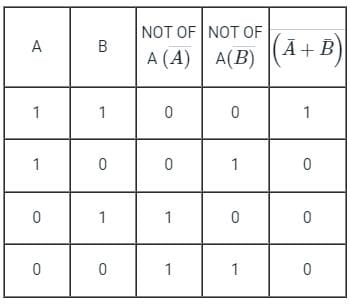This means the output will be always 1 when both inputs are 1, just like the AND gate.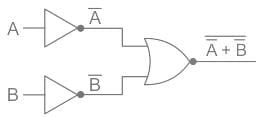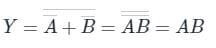Test: Logic Gates - 1 - Question 10

If A = 1 and B = 0, then in terms of Boolean algebra, A + B̅ =

Detailed Solution for Test: Logic Gates - 1 - Question 10

CONCEPT:

• The branch of algebra that deals with the values of the variables in the form of the truth values i.e., true and false are called Boolean algebra.
• True and false are usually denoted by 1 and 0 respectively.

Given that:
A = 1
B = 0
∴ B̅ = 1 (Since B̅ is reverse of B)
A + B̅ = 1 + 1 = 1 (Since A + A = A)
A + B̅ = 1 = A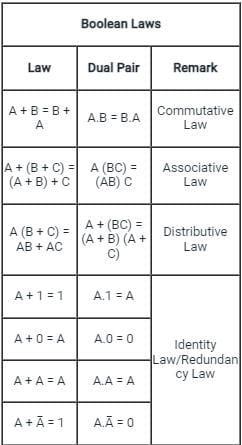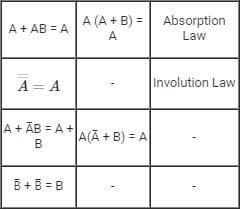## Digital Electronics

88 videos|71 docs|58 tests
 Use Code STAYHOME200 and get INR 200 additional OFF Use Coupon Code
Information about Test: Logic Gates - 1 Page
In this test you can find the Exam questions for Test: Logic Gates - 1 solved & explained in the simplest way possible. Besides giving Questions and answers for Test: Logic Gates - 1, EduRev gives you an ample number of Online tests for practice

## Digital Electronics

88 videos|71 docs|58 tests